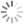Search Filters# 29 results tagged with Jewelry

Showing 1-29 of 29
Sort by     Items per page     Display Grid List RSS Feed
• Ornamental Jewelry Box 3D Model
3D Jewelry Box created in 3ds Max and zBrush.

Updated 2022-09-21 05:46:51 UTC
• Gucci pendant 2 3D Model
Gucci pendant 2

• Gucci pendant 3D Model
Gucci pendant

• Ice Age Character Sid 3D Model
Ice Age Character Sid

• dog shih tzu figure 2 3D Model
dog shih tzu figure 2

• beagle dog figure 3D Model
beagle dog figure

• cat figure 3 3D Model
cat figure 3

• elephant figure low poly 2 3D Model
elephant figure low poly 2

• dog shih tzu figure 3D Model
dog shih tzu figure

• panda figure 3 3D Model
panda figure 3

• panda figure 2 low poly 3D Model
panda figure 2 low poly

• panda figure low poly 3D Model
panda figure low poly

• dragon figure low poly 3D Model
dragon figure low poly

• girl figure low poly 3D Model
girl figure low poly

• elephant figure low poly 3D Model
elephant figure low poly

• sea horse figure low poly 3D Model
sea horse figure low poly

• Chowchow dog figure 2 3D Model
Chowchow dog figure 2

• bulldog figure 2 3D Model
bulldog figure 2

• Chowchow dog figure low poly 3D Model
Chowchow dog figure low poly

• bulldog figure low poly 3D Model
bulldog figure low poly

• low poly fish figure 3D Model
low poly fish figure

• parrot figure 2 3D Model
parrot figure 2

• Parrot figure 3D Model
Parrot figure

• Schnauzer dog figure 2 3D Model
Schnauzer dog figure 2

• cat figure 2 3D print model 3D Model
cat figure 2 3D print model

• baa-lamb jewelry 3D Model
pendant molded for the new year for the 3d printing